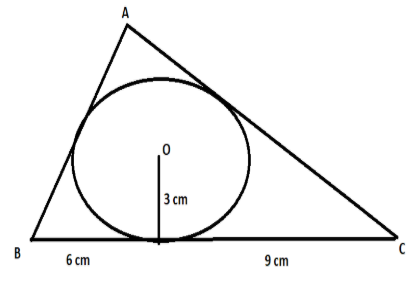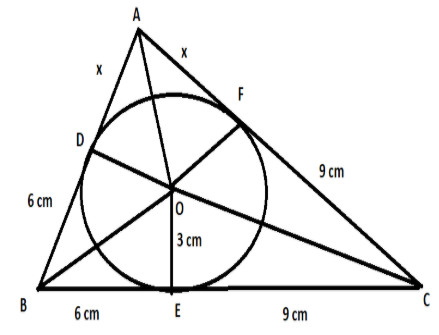Courses
Courses for Kids
Free study material
Free LIVE classes
MoreLIVE
Join Vedantu’s FREE Mastercalss

# In the figure given below, a $\Delta ABC$ is drawn to circumscribe a circle of radius 3 cm, such that the segments BD and DC are respectively of lengths 6 cm and 9 cm. If the area of $\Delta ABC$ is $54c{{m}^{2}}$, then find the lengths of sides AB and AC.Verified
361.8k+ views
Hint: In circles, we have a property that if two tangents are drawn from a point outside the circle to the circle, then the lengths of the two tangents from that point to the circle are equal. Also, if we draw a line from the centre of the circle to the point of contact of the tangent and the circle, the line from the centre will be perpendicular to the tangent. Using these properties, we can solve this question.

Before proceeding with the question, we must know all the properties of the circle that will be used to solve this question.
In circles, if we draw two tangents from an external point to the circle, the length of the two tangents are equal. $..............\left( 1 \right)$
Also, if we join the centre of the circle to the point of contact of the tangent and the circle, then this line will be perpendicular to the tangent. $..............\left( 2 \right)$
In this question, a $\Delta ABC$ is drawn to circumscribe a circle of radius 3 cm, such that the segments BD and DC are respectively of lengths 6 cm and 9 cm. Also, it is given that the area of this triangle ABC is equal to $54c{{m}^{2}}$. We are required to find the length of sides AB and AC.
Since a $\Delta ABC$ is drawn to circumscribe the circle, the sides AB, AC, BC will act as a tangent to the circle. Let us join all the points of contacts of tangents and the circle with the centre of the circle. Let us denote the point of contact with tangent AB as D, tangent BC as E and tangent AC as F. Also, let us join the centre of the circle O to vertices of the triangle A, B and C.Using property $\left( 1 \right)$, we can say CF = CE, BE = BD and AD = AF.
In the question, it is given BE = 6 cm and CE = 9 cm. Let us assume AD = x. Using above equation, we can say,
CF = CE = 9 cm
BE = BD = 6 cm
Also, it can be seen from the figure that,
Area of $\Delta ABC$ = area of $\Delta AOC$ + area of $\Delta BOC$ + area of $\Delta AOB...........\left( 3 \right)$
We have a formula that is of a triangle = $\dfrac{1}{2}$ (base)(height). From property $\left( 2 \right)$, we can see that OD, OE, OF are perpendicular to AB, AC, BC. So, OD, OE, OF can be taken as the heights of the 3 triangles. Also, as OD, OE, OF are the radius of the circles, their length will be equal to 3 cm. Using the formula of area of triangle,
Area of $\Delta AOC$= $\dfrac{1}{2}\left( 9+x \right)\left( 3 \right)$
Area of $\Delta BOC$= $\dfrac{1}{2}\left( 9+6 \right)\left( 3 \right)=\dfrac{45}{2}$
Area of $\Delta AOB$= $\dfrac{1}{2}\left( 6+x \right)\left( 3 \right)$
Also, it is given that area of triangle ABC is $54c{{m}^{2}}$.
Substituting these areas in equation $\left( 3 \right)$, we get,
\begin{align} & 54=\dfrac{1}{2}\left( 9+x \right)\left( 3 \right)+\dfrac{45}{2}+\dfrac{1}{2}\left( 6+x \right)\left( 3 \right) \\ & \Rightarrow 27+3x+45+18+3x=54\times 2 \\ & \Rightarrow 6x+90=108 \\ & \Rightarrow 6x=18 \\ & \Rightarrow x=3 \\ \end{align}
Hence, the length of the side AB = x + 6 = 3 + 6 = 9cm and the length of the side AC = x + 9 = 3 + 9 = 12 cm.

Note: It is a very common mistake that one may write the value of x as the answer. But in the question, we are asked to find the lengths of AB and AC. So, we have to find the length of AB and AC with the use of the obtained value of x and write these side lengths as the answer.
Last updated date: 24th Sep 2023
Total views: 361.8k
Views today: 4.61k